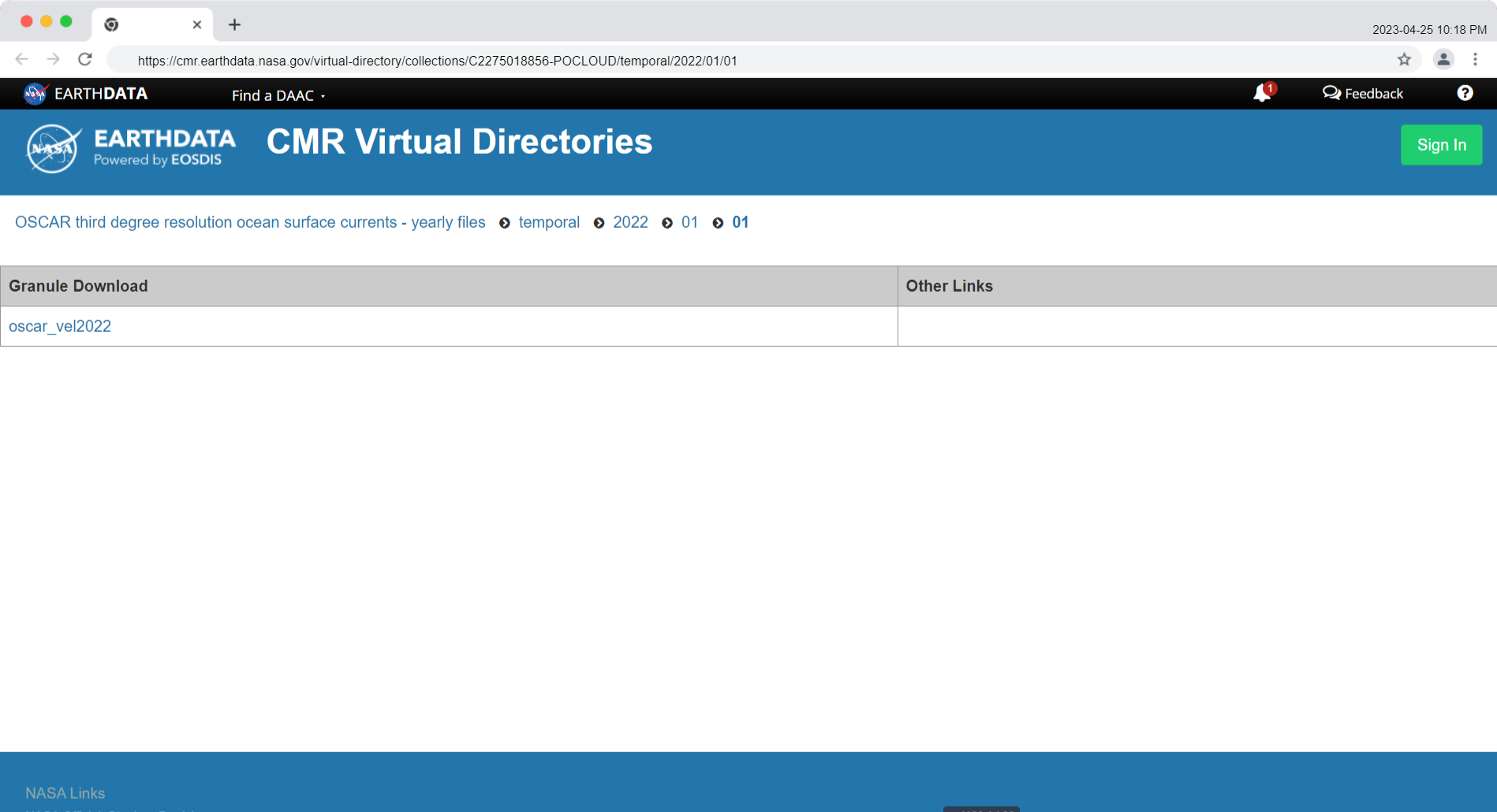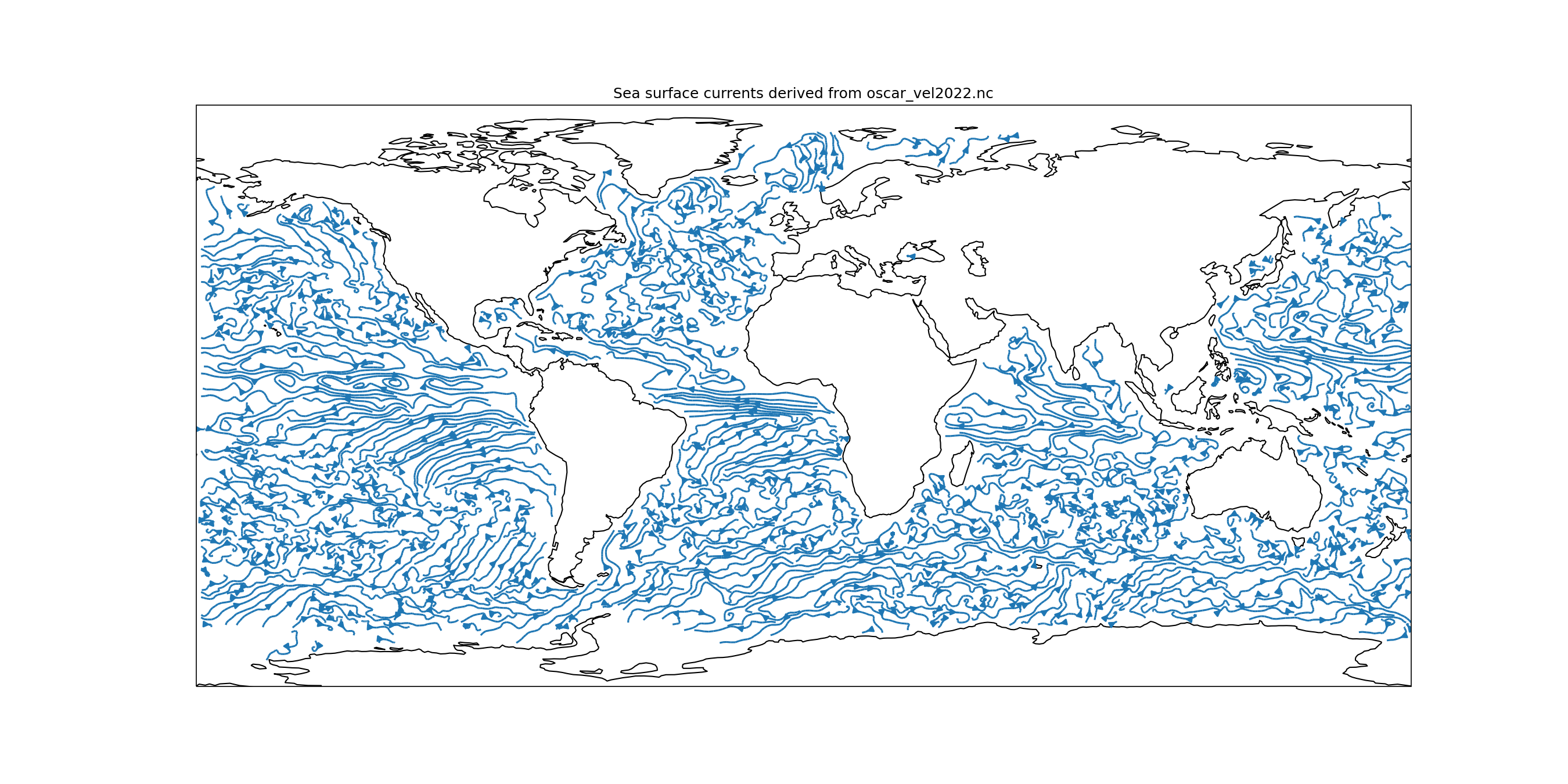# Preliminaries

perl create_netrc.pl


OSCAR third degree resolution ocean surface currents - yearly files

Short Name: OSCAR_L4_OC_third-deg_YEARLY# earthaccess

nsidc/earthaccess

We modify the example Python code for earthaccess:

#Import packages
import earthaccess

results = earthaccess.search_data(short_name="OSCAR_L4_OC_third-deg_YEARLY",
version="1",
cloud_hosted=True,
temporal = ("2022-01-01","2022-07-31"),
bounding_box = (-51.96423,68.10554,-48.71969,70.70529))



Then local_folder contains the single file, oscar_vel2022.nc.gz. We can use xarray directly with this file. But if we open the file with xarray two or more times, it becomes quicker to decompress it now. So we run gzip -d oscar_vel2022.nc.gz, and now local_folder contains the single file oscar_vel2022.nc. Then: 1

import xarray as xr

ds = xr.open_mfdataset("local_folder/oscar_vel2022.nc")


xarray.Dataset.info

ds.info()

xarray.Dataset {
dimensions:
time = 72 ;
year = 72 ;
depth = 1 ;
latitude = 481 ;
longitude = 1201 ;

variables:
datetime64[ns] time(time) ;
time:long_name = Day since 1992-10-05 00:00:00 ;
float32 year(year) ;
year:units = time in years ;
year:long_name = Time in fractional year ;
float32 depth(depth) ;
depth:units = meter ;
depth:long_name = Depth ;
float64 latitude(latitude) ;
latitude:units = degrees-north ;
latitude:long_name = Latitude ;
float64 longitude(longitude) ;
longitude:units = degrees-east ;
longitude:long_name = Longitude ;
float64 u(time, depth, latitude, longitude) ;
u:units = meter/sec ;
u:long_name = Ocean Surface Zonal Currents ;
float64 v(time, depth, latitude, longitude) ;
v:units = meter/sec ;
v:long_name = Ocean Surface Meridional Currents ;
float64 um(time, depth, latitude, longitude) ;
um:units = meter/sec ;
um:long_name = Ocean Surface Zonal Currents Maximum Mask ;
float64 vm(time, depth, latitude, longitude) ;
vm:units = meter/sec ;
vm:long_name = Ocean Surface Meridional Currents Maximum Mask ;

// global attributes:
:VARIABLE = Ocean Surface Currents ;
:DATATYPE = 1/72 YEAR Interval ;
:DATASUBTYPE = unfiltered ;
:GEORANGE = 20 to 420 -80 to 80 ;
:PERIOD = Jan.01,2022 to Dec.26,2022 ;
:year = 2022 ;
:description = OSCAR Third Degree Sea Surface Velocity ;
:CREATION_DATE = 02:21 06-Feb-2023 ;
:version = 2009.0 ;
:source = Gary Lagerloef, ESR (lager@esr.org) and Kathleen Dohan, ESR (kdohan@esr.org) ;
:contact = Kathleen Dohan (kdohan@esr.org) or John T. Gunn (gunn@esr.org) ;
:company = Earth & Space Research, Seattle, WA ;
:reference = Bonjean F. and G.S.E. Lagerloef, 2002 ,Diagnostic model and analysis of the surface currents in the tropical Pacific ocean, J. Phys. Oceanogr., 32, 2,938-2,954 ;
:note1 = Maximum Mask velocity is the geostrophic component at all points + any concurrent Ekman and buoyancy components ;
:note2 = Longitude extends from 20 E to 420 E to avoid a break in major ocean basins. Data repeats in overlap region. ;
}


Next:

import numpy as np
import cartopy.crs as ccrs
import matplotlib.pyplot as plt

plt.figure(figsize=(18, 9))

ax = plt.axes(projection=ccrs.PlateCarree()) # plate carrée projection

dec = 2

lon = ds.longitude.values[::dec]

lon[lon > 180] = lon[lon > 180] - 360

mymap = plt.streamplot(
lon,
ds.latitude.values[::dec],
ds.u.values[0, 0, ::dec, ::dec],
ds.v.values[0, 0, ::dec, ::dec],
8,
transform = ccrs.PlateCarree()
)

ax.coastlines()

plt.title('Sea surface currents derived from OSCAR')

plt.savefig("currents18x9E.png", dpi=150)

plt.show()


OSCAR 2022 streamplot:My so-far favorite resources for xarray:

Xarray Tutorial

Xarray Tutorial | Pangeo

Physics of the Climate System | Fabien Maussion